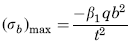Related Resources: calculators

### Uniform Decreasing Loading Flat Plate Stress and Deflection Equation and Calculator

Flat Rectangular Plate; all edges fixed. Uniform Decreasing Loading Parallel to Side b Stress and Deflection Equation and Calculator.

Per. Roarks Formulas for Stress and Strain for flat plates with straight boundaries and constant thickness

Rectangular Plate; all edges fixed. Uniform Decreasing Loading Parallel to Side b.

 Rectangular plate; all Edges FixedUniformly decreasing load parallel to side bStress at x = 0, z = 0Stress at x = 0, z = 0.4bandStress at x = 0, z = bStress at x = ± a/2, z = 0.45bDeflection at center:Symbols used:
E = Modulus of Elasticity (lbs/in2)
q = Total load or force to Plate (lbs/in2)
v = Poisson’s ratio (assumed to be 0.3)
t = plate thickness, (in)
a = plate length, (in)
b = plate width, (in)
σb = stress, (lbs/in2)
γmax = Deflection (in)
β1,2, 3, 4, 5 = Constant From Table A
α = Constant From Table A

Table A

 a/b 0.6 0.8 1 1.2 1.4 1.6 1.8 2 β1 0.1132 0.1778 0.2365 0.2777 0.3004 0.3092 0.31 0.3068 β2 0.041 0.0633 0.0869 0.1038 0.1128 0.1255 0.1157 0.1148 β3 0.0637 0.0688 0.0762 0.0715 0.061 0.0509 0.0415 0.0356 β4 0.0206 0.0497 0.0898 0.1249 0.1482 0.1615 0.168 0.1709 β5 0.1304 0.1436 0.1686 0.18 0.1845 0.1874 0.1902 0.1908 α 0.0016 0.0047 0.0074 0.0097 0.0113 0.0126 0.0133 0.0136

Reference:

Roarks Formulas for Stress and Strain, 7th Edition